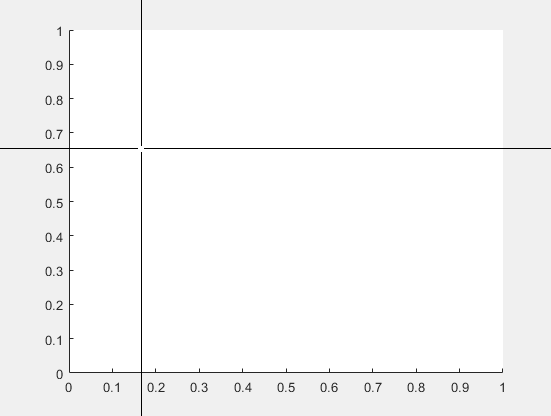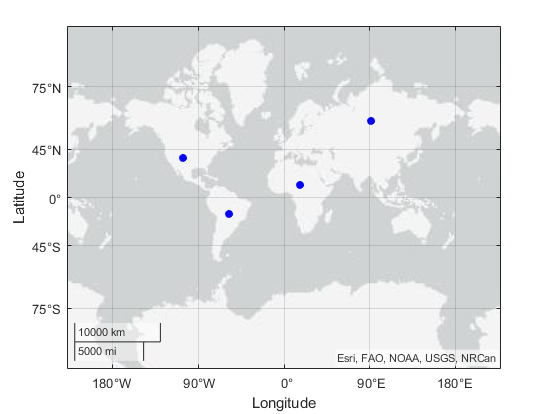# ginput

Identify axes coordinates

## Syntax

``[x,y] = ginput(n)``
``[x,y] = ginput``
``[x,y,button] = ginput(___)``

## Description

example

````[x,y] = ginput(n)` allows you to identify the coordinates of `n` points within Cartesian, polar, or geographic axes. To choose a point, move your cursor to the desired location and press either a mouse button or a key on the keyboard. Press the Return key to stop before all `n` points are selected. MATLAB® returns the coordinates of your selected points. If there are no axes, calling `ginput` creates a Cartesian axes object.```

example

````[x,y] = ginput` allows you to select an unlimited number of points until you press the Return key.```

example

````[x,y,button] = ginput(___)` also returns the mouse button or key on the keyboard used to select each point.```

## Examples

collapse all

Identify four points in a set of axes using `ginput`. To select each point, move the cursor to your desired location and press a mouse button or key.

`[x,y] = ginput(4)````x = 0.3699 0.4425 0.5450 0.6130 y = 0.6690 0.5605 0.4719 0.6025```

Plot the points.

`plot(x,y);`Identify five coordinates in a set of axes using `ginput`. To select each point, move the cursor to your desired location and press a mouse button, lowercase letter, uppercase letter, number, or the space bar. Return the mouse buttons or ASCII numbers of the keys used to select each point.

```[x,y,button] = ginput(5); button ```
```button = 3 104 32 51 82```

In this case, the coordinates were identified using the right mouse button (3), the lowercase letter h (104), the space bar (32), the number 3 (51), and the uppercase letter R (82).

Create geographic axes and identify the latitude and longitude coordinates of four points. Then, plot the points that you identify.

```geoaxes [lat,lon] = ginput(4);```Use the `hold on` command and the `geolimits` function to maintain the map limits. Plot the identified points.

```hold on geolimits('manual') geoscatter(lat,lon,'filled','b')```## Input Arguments

collapse all

Number of points to identify, specified as a positive integer.

## Output Arguments

collapse all

First components of the identified coordinates, returned as a scalar or column vector.

• For Cartesian axes, the values are `x`-coordinates.

• For polar axes, the values are theta angles. The theta angles are in the range `[tmin, tmin+2*pi]`, where `tmin` is the first value of the `ThetaLim` vector of the polar axes. The angles are measured from the location of the zero angle in the direction specified by the `ThetaDir` property. The location of the zero angle is specified by the `ThetaZeroLocation` property.

• For geographic axes, the values are latitudes. Positive values correspond to north and negative values correspond to south.

If you choose points outside the axes limits, the values are relative to the origin for Cartesian and geographic axes. For polar axes, the values are relative to the `ThetaZeroLocation`.

Second components of the identified coordinates, returned as a scalar or column vector.

• For Cartesian axes , the values are `y`-coordinates.

• For polar axes, the values are radii. Each radius value is greater than or equal to `rmin`, where `rmin` is the first value in the `RLim` vector of the polar axes.

• For geographic axes, the values are longitudes. Positive values correspond to locations east of the origin and negative values correspond to locations west of the origin.

If you choose points outside the axes limits, values are still returned relative to the axes origin.

Keys or mouse buttons used to identify points, returned as a scalar or column vector. Mouse buttons are indicated by 1 for the left button, 2 for the middle, and 3 for the right. Double-clicks are indicated by two values. The first value is 1 for the left mouse button, 2 for the middle, and 3 for the right. The second value is 1.

Keys on the keyboard are indicated by their corresponding ASCII numbers. Taps on a screen are indicated by 1.

For example, call `ginput`, click using the middle mouse button, double-click using the right mouse button, and then press Enter. The output `button` is a vector containing `2`, `3`, and `1`.

`[~,~,button] = ginput`
```button = 2 3 1```

## Version History

Introduced before R2006a